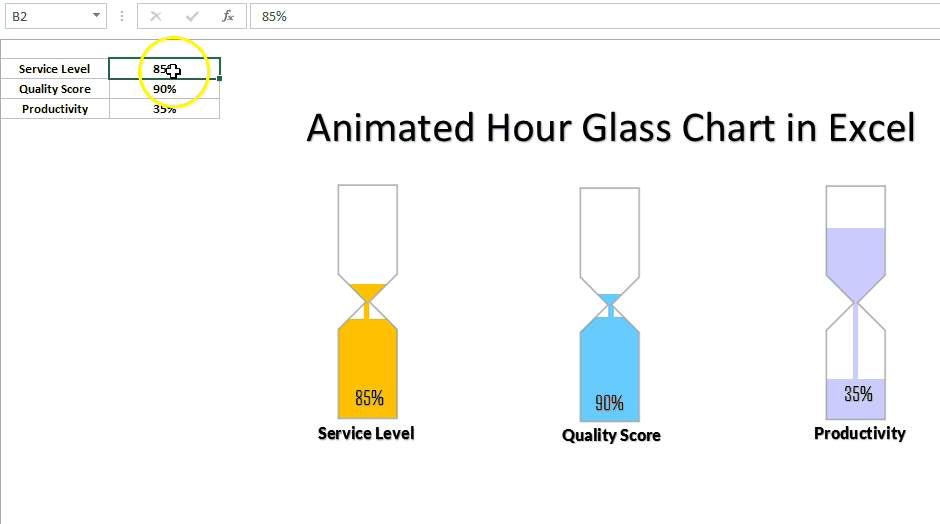Home>Blogs>Charts and Visualization>Info-graphics: Animated Hour Glass Chart in Excel

# Info-graphics: Animated Hour Glass Chart in Excel

Animated Hour Glass Chart has been designed to display the KPI metrics like – Service Level, Quality Score, and Productivity etc. Whenever the value of metric will be changes it will show an animation like below given image.### We have put the below given code on Worksheet Change event to show the animation

```Option Explicit

Private Sub Worksheet_Change(ByVal Target As Range)

Dim i As Integer

For i = 0 To Int(Target.Value * 100)

VBA.DoEvents

ActiveSheet.Range("N1").Value = i / 100

Next i

ActiveSheet.Range("N1").Value = Target.Value

End If

For i = 0 To Int(Target.Value * 100)

VBA.DoEvents

ActiveSheet.Range("N2").Value = i / 100

Next i

ActiveSheet.Range("N2").Value = Target.Value

End If

For i = 0 To Int(Target.Value * 100)

VBA.DoEvents

ActiveSheet.Range("N3").Value = i / 100

Next i

ActiveSheet.Range("N3").Value = Target.Value

End If

End Sub```